# 零知识证明 Learn by Coding：libsnark 入门篇

https://www.interchains.cc/21594.html

### 零知识证明 Learn by Coding：libsnark 入门篇

libsnark 是目前实现 zk-SNARKs 电路最重要的框架，在众多私密交易或隐私计算相关项目间广泛应用，其中最著名当然要数 Zcash。Zcash 在 Sapling 版本升级前一直使用 libsnark 来实现电路（之后才替换为 bellman）。毫不夸张地说，libsnark 支撑并促进了 zk-SNARKs 技术的首次大规模应用，填补了零知识证明技术从最新理论到工程实现间的空缺。

## 1. zk-SNARKs 和 libsnark 背景简介

zk-SNARKs 能应用到很多场景，比如隐私保护、区块链blockchain扩容、可验证计算等。本文不介绍 zk-SNARKS 和零知识证明的理论细节，不熟悉或想深入了解的同学可阅读其他文章或论文。

• https://medium.com/@VitalikButerin/exploring-elliptic-curve-pairings-c73c1864e627
• https://medium.com/@VitalikButerin/zk-snarks-under-the-hood-b33151a013f6

• 「深入浅出零知识证明之zk-SNARKs」：https://www.yuque.com/u428635/scg32w/edmn74
• 「浅谈零知识证明之二：简短无交互证明（SNARK）」：(https://learnblockchain.cn/article/352)
• 「探索零知识证明」系列：https://learnblockchain.cn/2019/08/01/learning-zkp
• 「从零开始学习 zk-SNARK」系列：https://learnblockchain.cn/article/287
• 「零知识证明学习资源汇总」：https://learnblockchain.cn/2019/11/08/zkp-info

• [GGPR13] Quadratic span programs and succinct NIZKs without PCPs , Rosario Gennaro, Craig Gentry, Bryan Parno, Mariana Raykova, EUROCRYPT 2013
• [PGHR13] Pinocchio: Nearly Practical Verifiable Computation , Bryan Parno, Craig Gentry, Jon Howell, Mariana Raykova, IEEE Symposium on Security and Privacy (Oakland) 2013
• [BCGTV13] SNARKs for C: Verifying Program Executions Succinctly and in Zero Knowledge , Eli Ben-Sasson, Alessandro Chiesa, Daniel Genkin, Eran Tromer, Madars Virza, CRYPTO 2013
• [BCIOP13] Succinct non-interactive arguments via linear interactive Proofs , Nir Bitansky, Alessandro Chiesa, Yuval Ishai, Rafail Ostrovsky, Omer Paneth, Theory of Cryptography Conference 2013
• [BCTV14a] Succinct non-interactive zero knowledge for a von Neumann architecture , Eli Ben-Sasson, Alessandro Chiesa, Eran Tromer, Madars Virza, USENIX Security 2014
• [BCTV14b] Scalable succinct non-interactive arguments via cycles of elliptic curves , Eli Ben-Sasson, Alessandro Chiesa, Eran Tromer, Madars Virza, CRYPTO 2014
• [Groth16] On the Size of Pairing-based Non-interactive Arguments , Jens Groth, EUROCRYPT 2016

libsnark 的开发者们亦是这个领域内顶尖的学者或研究牛人，如 Eran Tromer 更是以上多篇论文的共同作者。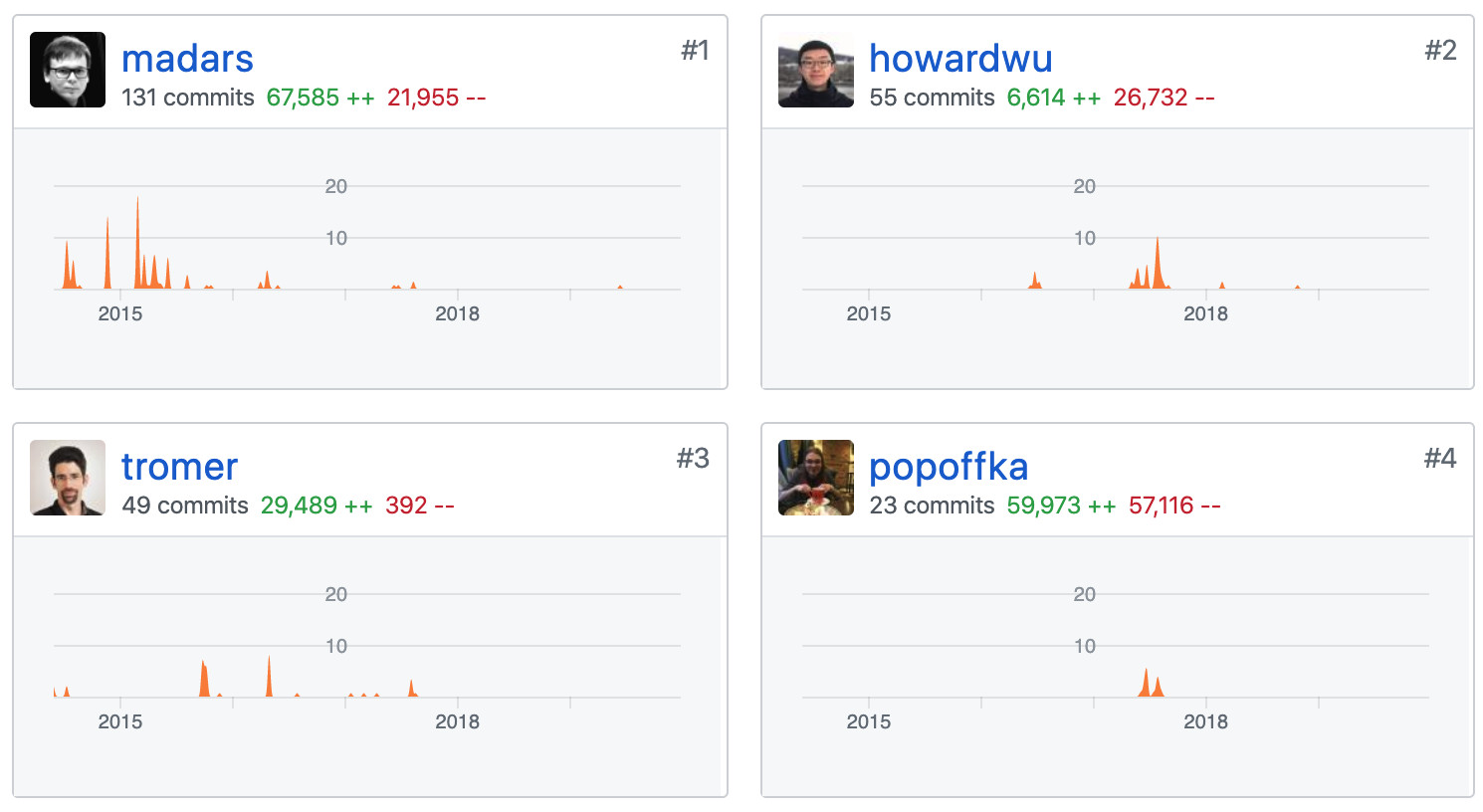扎实的理论基础和工程能力，让 libsnark 的作者们能够化繁为简，将形如下图的高深理论和复杂公式逐一实现，高度工程化地抽象出简洁的接口供广大开发者方便地调用。向这些将非凡的理论研究推广至更大规模应用的先锋们致敬。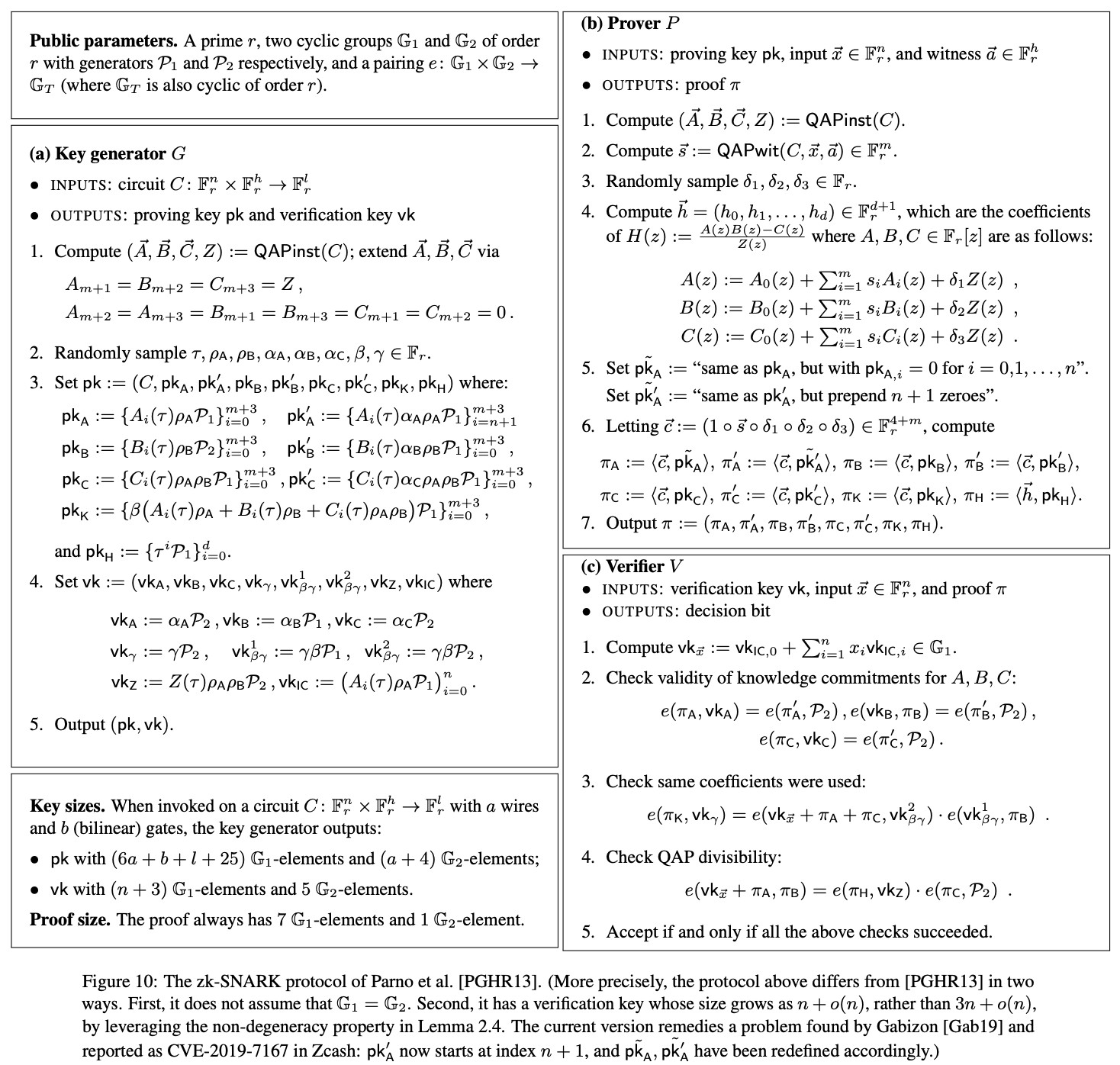下图是 libsnark 的模块总览图，摘自 libsnark 代码贡献量第一作者 Madars Virza 在 MIT 的博士论文。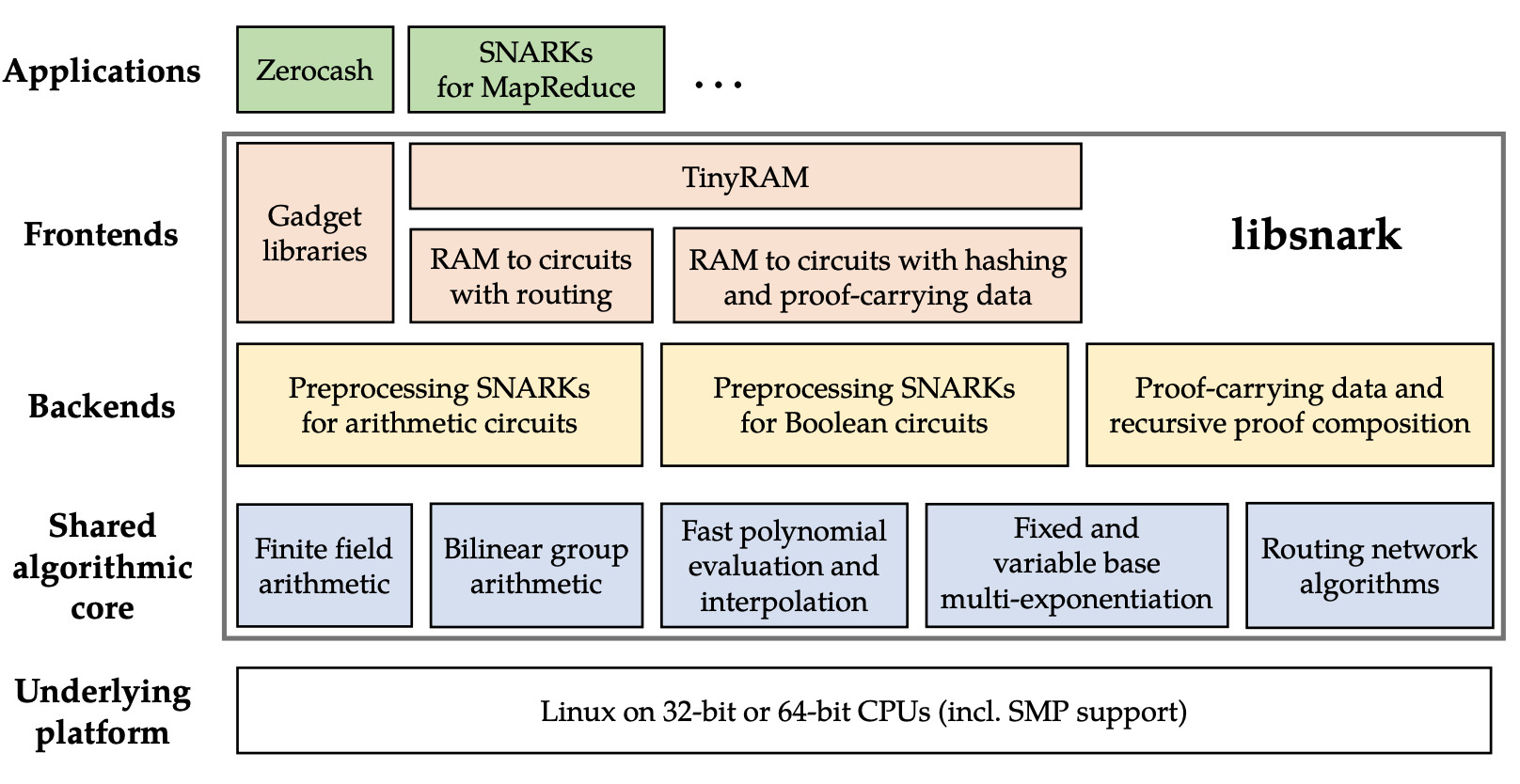libsnark 框架提供了多个通用证明系统的实现，其中使用较多的是 BCTV14a 和 Groth16。

• zk_proof_systems/ppzksnark/r1cs_ppzksnark 对应的是 BCTV14a
• zk_proof_systems/ppzksnark/r1cs_gg_ppzksnark 对应的是 Groth16

## 2. 基本原理与步骤

1. 将待证明的命题表达为 R1CS (Rank One Constraint System)
2. 使用生成算法（G）为该命题生成公共参数
3. 使用证明算法（P）生成 R1CS 可满足性的证明
4. 使用验证算法（V）来验证证明

``function C(x, out) {   return ( x^3 + x + 5 == out ); }``

``lambda &lt;- random()  (pk, vk) = G(C, lambda)``

``proof = P(pk, out, x)``

``V(vk, out, proof) ?= true``

## 3. 搭建 zk-SNARKs 应用开发环境

``git clone https://github.com/sec-bit/libsnark_abc.git``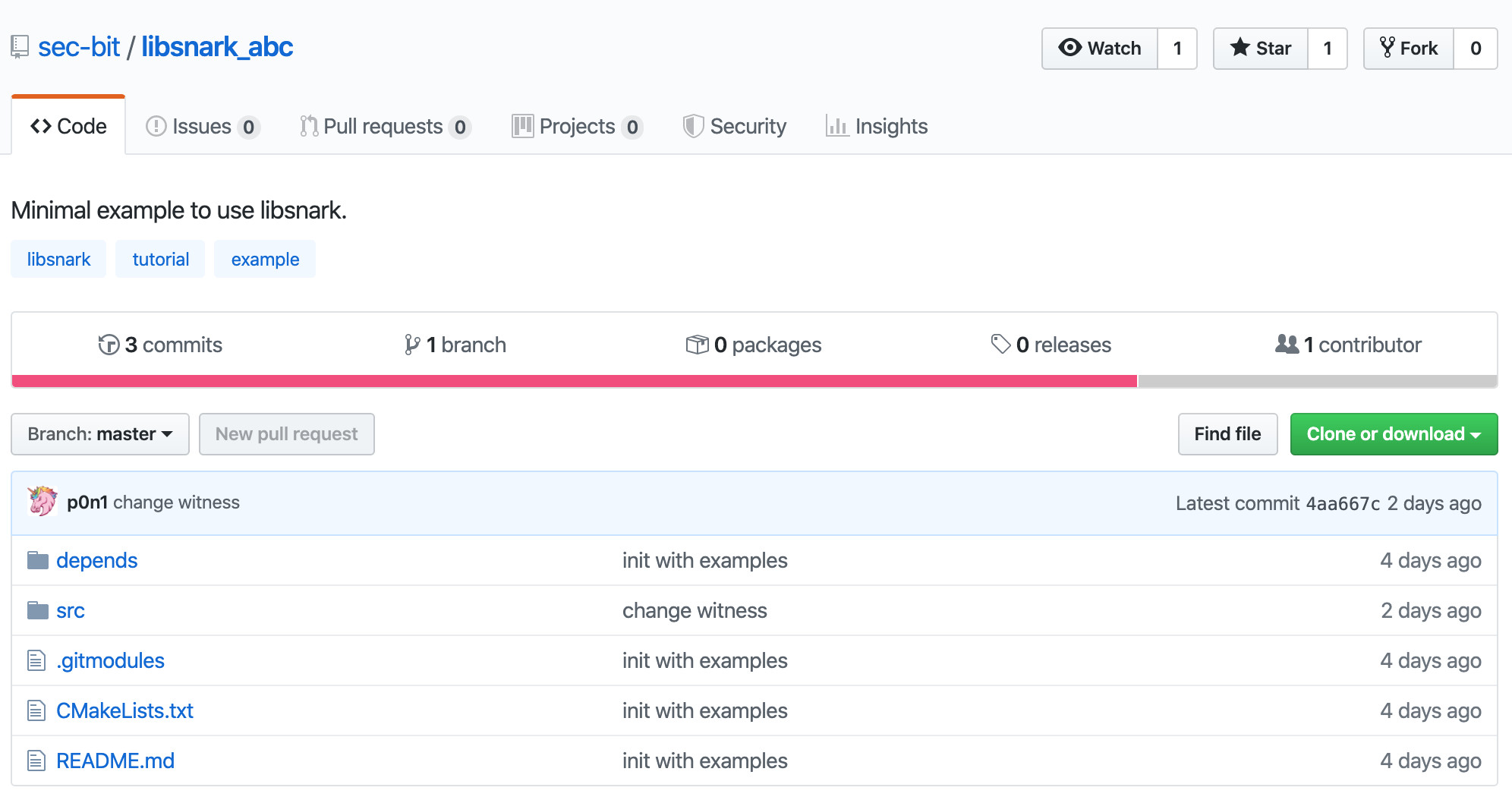通过 git submodule 拉取 libsnark 代码。

``cd libsnark_abc git submodule update --init --recursive``

``sudo apt-get install build-essential cmake git libgmp3-dev libprocps4-dev python-markdown libboost-all-dev libssl-dev``

``mkdir build && cd build && cmake ..``

``mkdir build && cd build && CPPFLAGS=-I/usr/local/opt/openssl/include LDFLAGS=-L/usr/local/opt/openssl/lib PKG_CONFIG_PATH=/usr/local/opt/openssl/lib/pkgconfig cmake -DWITH_PROCPS=OFF -DWITH_SUPERCOP=OFF ..``

``make``

``main range test``

``./src/main``

## 4. 理解示例代码

``r1cs_gg_ppzksnark_keypair&lt;ppT> keypair = r1cs_gg_ppzksnark_generator&lt;ppT>(example.constraint_system); r1cs_gg_ppzksnark_processed_verification_key&lt;ppT> pvk = r1cs_gg_ppzksnark_verifier_process_vk&lt;ppT>(keypair.vk); r1cs_gg_ppzksnark_proof&lt;ppT> proof = r1cs_gg_ppzksnark_prover&lt;ppT>(keypair.pk, example.primary_input, example.auxiliary_input); const bool ans = r1cs_gg_ppzksnark_verifier_strong_IC&lt;ppT>(keypair.vk, example.primary_input, proof); const bool ans2 = r1cs_gg_ppzksnark_online_verifier_strong_IC&lt;ppT>(pvk, example.primary_input, proof);``

``#include &lt;libsnark/common/default_types/r1cs_gg_ppzksnark_pp.hpp> #include &lt;libsnark/zk_proof_systems/ppzksnark/r1cs_gg_ppzksnark/r1cs_gg_ppzksnark.hpp> #include &lt;libsnark/gadgetlib1/pb_variable.hpp>``

``typedef libff::Fr&lt;default_r1cs_gg_ppzksnark_pp> FieldT; default_r1cs_gg_ppzksnark_pp::init_public_params();``

``x * x = sym_1 sym_1 * x = y y + x = sym_2 sym_2 + 5 = out``

``// Create protoboard protoboard&lt;FieldT> pb; // Define variables pb_variable&lt;FieldT> x; pb_variable&lt;FieldT> sym_1; pb_variable&lt;FieldT> y; pb_variable&lt;FieldT> sym_2; pb_variable&lt;FieldT> out;``

``out.allocate(pb, "out"); x.allocate(pb, "x"); sym_1.allocate(pb, "sym_1"); y.allocate(pb, "y"); sym_2.allocate(pb, "sym_2"); pb.set_input_sizes(1);``

``// x*x = sym_1 pb.add_r1cs_constraint(r1cs_constraint&lt;FieldT>(x, x, sym_1)); // sym_1 * x = y pb.add_r1cs_constraint(r1cs_constraint&lt;FieldT>(sym_1, x, y)); // y + x = sym_2 pb.add_r1cs_constraint(r1cs_constraint&lt;FieldT>(y + x, 1, sym_2)); // sym_2 + 5 = ~out pb.add_r1cs_constraint(r1cs_constraint&lt;FieldT>(sym_2 + 5, 1, out));``

``const r1cs_constraint_system&lt;FieldT> constraint_system = pb.get_constraint_system(); const r1cs_gg_ppzksnark_keypair&lt;default_r1cs_gg_ppzksnark_pp> keypair = r1cs_gg_ppzksnark_generator&lt;default_r1cs_gg_ppzksnark_pp>(constraint_system);``

``pb.val(out) = 35; pb.val(x) = 3; pb.val(sym_1) = 9; pb.val(y) = 27; pb.val(sym_2) = 30;``

``const r1cs_gg_ppzksnark_proof&lt;default_r1cs_gg_ppzksnark_pp> proof = r1cs_gg_ppzksnark_prover&lt;default_r1cs_gg_ppzksnark_pp>(keypair.pk, pb.primary_input(), pb.auxiliary_input());``

``bool verified = r1cs_gg_ppzksnark_verifier_strong_IC&lt;default_r1cs_gg_ppzksnark_pp>(keypair.vk, pb.primary_input(), proof);``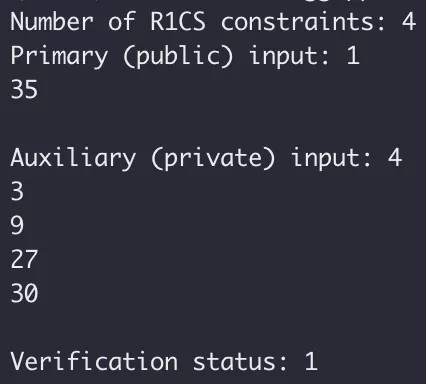## 5 再次上手实践

zk-SNARKs 电路开发的主要工作量和难点在于如何用代码“精确”地描述命题中的所有约束。一旦描述不“精确”，则要么是漏掉约束、要么是写错约束，最终电路想要证明的内容则会与原命题相差甚远。上一节的例子只涉及简单的乘法和加法，与 r1cs_constraint 最基本的形式一致，因此约束的表达相对容易。除此之外几乎所有的约束都不是很直观，作为初学者很难正确地描述约束细节。

``comparison_gadget(protoboard&lt;FieldT>& pb,                     const size_t n,                     const pb_linear_combination&lt;FieldT> &A,                     const pb_linear_combination&lt;FieldT> &B,                     const pb_variable&lt;FieldT> &less,                     const pb_variable&lt;FieldT> &less_or_eq,                     const std::string &annotation_prefix="")``

``protoboard&lt;FieldT> pb; pb_variable&lt;FieldT> x, max; pb_variable&lt;FieldT> less, less_or_eq; x.allocate(pb, "x"); max.allocate(pb, "max"); pb.val(max)= 60;``

``comparison_gadget&lt;FieldT> cmp(pb, 10, x, max, less, less_or_eq, "cmp"); cmp.generate_r1cs_constraints(); pb.add_r1cs_constraint(r1cs_constraint&lt;FieldT>(less, 1, FieldT::one()));``

``// Add witness values pb.val(x) = 18; // secret cmp.generate_r1cs_witness();``

## 7 附录

### main.cpp

``#include &lt;libff/common/default_types/ec_pp.hpp> #include &lt;libsnark/common/default_types/r1cs_gg_ppzksnark_pp.hpp> #include &lt;libsnark/relations/constraint_satisfaction_problems/r1cs/examples/r1cs_examples.hpp> #include &lt;libsnark/zk_proof_systems/ppzksnark/r1cs_gg_ppzksnark/r1cs_gg_ppzksnark.hpp> using namespace libsnark; /**  * The code below provides an example of all stages of running a R1CS GG-ppzkSNARK.  *  * Of course, in a real-life scenario, we would have three distinct entities,  * mangled into one in the demonstration below. The three entities are as follows.  * (1) The "generator", which runs the ppzkSNARK generator on input a given  *     constraint system CS to create a proving and a verification key for CS.  * (2) The "prover", which runs the ppzkSNARK prover on input the proving key,  *     a primary input for CS, and an auxiliary input for CS.  * (3) The "verifier", which runs the ppzkSNARK verifier on input the verification key,  *     a primary input for CS, and a proof.  */ template&lt;typename ppT> bool run_r1cs_gg_ppzksnark(const r1cs_example&lt;libff::Fr&lt;ppT> > &example) {     libff::print_header("R1CS GG-ppzkSNARK Generator");     r1cs_gg_ppzksnark_keypair&lt;ppT> keypair = r1cs_gg_ppzksnark_generator&lt;ppT>(example.constraint_system);     printf("n"); libff::print_indent(); libff::print_mem("after generator");     libff::print_header("Preprocess verification key");     r1cs_gg_ppzksnark_processed_verification_key&lt;ppT> pvk = r1cs_gg_ppzksnark_verifier_process_vk&lt;ppT>(keypair.vk);     libff::print_header("R1CS GG-ppzkSNARK Prover");     r1cs_gg_ppzksnark_proof&lt;ppT> proof = r1cs_gg_ppzksnark_prover&lt;ppT>(keypair.pk, example.primary_input, example.auxiliary_input);     printf("n"); libff::print_indent(); libff::print_mem("after prover");     libff::print_header("R1CS GG-ppzkSNARK Verifier");     const bool ans = r1cs_gg_ppzksnark_verifier_strong_IC&lt;ppT>(keypair.vk, example.primary_input, proof);     printf("n"); libff::print_indent(); libff::print_mem("after verifier");     printf("* The verification result is: %sn", (ans ? "PASS" : "FAIL"));     libff::print_header("R1CS GG-ppzkSNARK Online Verifier");     const bool ans2 = r1cs_gg_ppzksnark_online_verifier_strong_IC&lt;ppT>(pvk, example.primary_input, proof);     assert(ans == ans2);     return ans; } template&lt;typename ppT> void test_r1cs_gg_ppzksnark(size_t num_constraints, size_t input_size) {     r1cs_example&lt;libff::Fr&lt;ppT> > example = generate_r1cs_example_with_binary_input&lt;libff::Fr&lt;ppT> >(num_constraints, input_size);     const bool bit = run_r1cs_gg_ppzksnark&lt;ppT>(example);     assert(bit); } int main () {     default_r1cs_gg_ppzksnark_pp::init_public_params();     test_r1cs_gg_ppzksnark&lt;default_r1cs_gg_ppzksnark_pp>(1000, 100);     return 0; }``

### test.cpp

``#include &lt;libsnark/common/default_types/r1cs_gg_ppzksnark_pp.hpp> #include &lt;libsnark/zk_proof_systems/ppzksnark/r1cs_gg_ppzksnark/r1cs_gg_ppzksnark.hpp> #include &lt;libsnark/gadgetlib1/pb_variable.hpp> using namespace libsnark; using namespace std; int main () {     typedef libff::Fr&lt;default_r1cs_gg_ppzksnark_pp> FieldT;     // Initialize the curve parameters     default_r1cs_gg_ppzksnark_pp::init_public_params();      // Create protoboard     protoboard&lt;FieldT> pb;     // Define variables     pb_variable&lt;FieldT> x;     pb_variable&lt;FieldT> sym_1;     pb_variable&lt;FieldT> y;     pb_variable&lt;FieldT> sym_2;     pb_variable&lt;FieldT> out;     // Allocate variables to protoboard     // The strings (like "x") are only for debugging purposes         out.allocate(pb, "out");     x.allocate(pb, "x");     sym_1.allocate(pb, "sym_1");     y.allocate(pb, "y");     sym_2.allocate(pb, "sym_2");     // This sets up the protoboard variables     // so that the first one (out) represents the public     // input and the rest is private input     pb.set_input_sizes(1);     // Add R1CS constraints to protoboard     // x*x = sym_1     pb.add_r1cs_constraint(r1cs_constraint&lt;FieldT>(x, x, sym_1));     // sym_1 * x = y     pb.add_r1cs_constraint(r1cs_constraint&lt;FieldT>(sym_1, x, y));     // y + x = sym_2     pb.add_r1cs_constraint(r1cs_constraint&lt;FieldT>(y + x, 1, sym_2));     // sym_2 + 5 = ~out     pb.add_r1cs_constraint(r1cs_constraint&lt;FieldT>(sym_2 + 5, 1, out));      const r1cs_constraint_system&lt;FieldT> constraint_system = pb.get_constraint_system();     // generate keypair     const r1cs_gg_ppzksnark_keypair&lt;default_r1cs_gg_ppzksnark_pp> keypair = r1cs_gg_ppzksnark_generator&lt;default_r1cs_gg_ppzksnark_pp>(constraint_system);     // Add public input and witness values     pb.val(out) = 35;     pb.val(x) = 3;     pb.val(sym_1) = 9;     pb.val(y) = 27;     pb.val(sym_2) = 30;     // generate proof     const r1cs_gg_ppzksnark_proof&lt;default_r1cs_gg_ppzksnark_pp> proof = r1cs_gg_ppzksnark_prover&lt;default_r1cs_gg_ppzksnark_pp>(keypair.pk, pb.primary_input(), pb.auxiliary_input());     // verify     bool verified = r1cs_gg_ppzksnark_verifier_strong_IC&lt;default_r1cs_gg_ppzksnark_pp>(keypair.vk, pb.primary_input(), proof);     cout &lt;&lt; "Number of R1CS constraints: " &lt;&lt; constraint_system.num_constraints() &lt;&lt; endl;     cout &lt;&lt; "Primary (public) input: " &lt;&lt; pb.primary_input() &lt;&lt; endl;     cout &lt;&lt; "Auxiliary (private) input: " &lt;&lt; pb.auxiliary_input() &lt;&lt; endl;     cout &lt;&lt; "Verification status: " &lt;&lt; verified &lt;&lt; endl; }``

### range.cpp

``#include &lt;libsnark/common/default_types/r1cs_gg_ppzksnark_pp.hpp> #include &lt;libsnark/zk_proof_systems/ppzksnark/r1cs_gg_ppzksnark/r1cs_gg_ppzksnark.hpp> #include &lt;libsnark/gadgetlib1/pb_variable.hpp> #include &lt;libsnark/gadgetlib1/gadgets/basic_gadgets.hpp> using namespace libsnark; using namespace std; int main () {     typedef libff::Fr&lt;default_r1cs_gg_ppzksnark_pp> FieldT;     // Initialize the curve parameters     default_r1cs_gg_ppzksnark_pp::init_public_params();      // Create protoboard     protoboard&lt;FieldT> pb;     pb_variable&lt;FieldT> x, max;     pb_variable&lt;FieldT> less, less_or_eq;     x.allocate(pb, "x");     max.allocate(pb, "max");      pb.val(max)= 60;     comparison_gadget&lt;FieldT> cmp(pb, 10, x, max, less, less_or_eq, "cmp");     cmp.generate_r1cs_constraints();     pb.add_r1cs_constraint(r1cs_constraint&lt;FieldT>(less, 1, FieldT::one()));     const r1cs_constraint_system&lt;FieldT> constraint_system = pb.get_constraint_system();     // generate keypair     const r1cs_gg_ppzksnark_keypair&lt;default_r1cs_gg_ppzksnark_pp> keypair = r1cs_gg_ppzksnark_generator&lt;default_r1cs_gg_ppzksnark_pp>(constraint_system);     // Add witness values     pb.val(x) = 18; // secret     cmp.generate_r1cs_witness();     // generate proof     const r1cs_gg_ppzksnark_proof&lt;default_r1cs_gg_ppzksnark_pp> proof = r1cs_gg_ppzksnark_prover&lt;default_r1cs_gg_ppzksnark_pp>(keypair.pk, pb.primary_input(), pb.auxiliary_input());     // verify     bool verified = r1cs_gg_ppzksnark_verifier_strong_IC&lt;default_r1cs_gg_ppzksnark_pp>(keypair.vk, pb.primary_input(), proof);     cout &lt;&lt; "Number of R1CS constraints: " &lt;&lt; constraint_system.num_constraints() &lt;&lt; endl;     cout &lt;&lt; "Primary (public) input: " &lt;&lt; pb.primary_input() &lt;&lt; endl;     cout &lt;&lt; "Auxiliary (private) input: " &lt;&lt; pb.auxiliary_input() &lt;&lt; endl;     cout &lt;&lt; "Verification status: " &lt;&lt; verified &lt;&lt; endl; }``